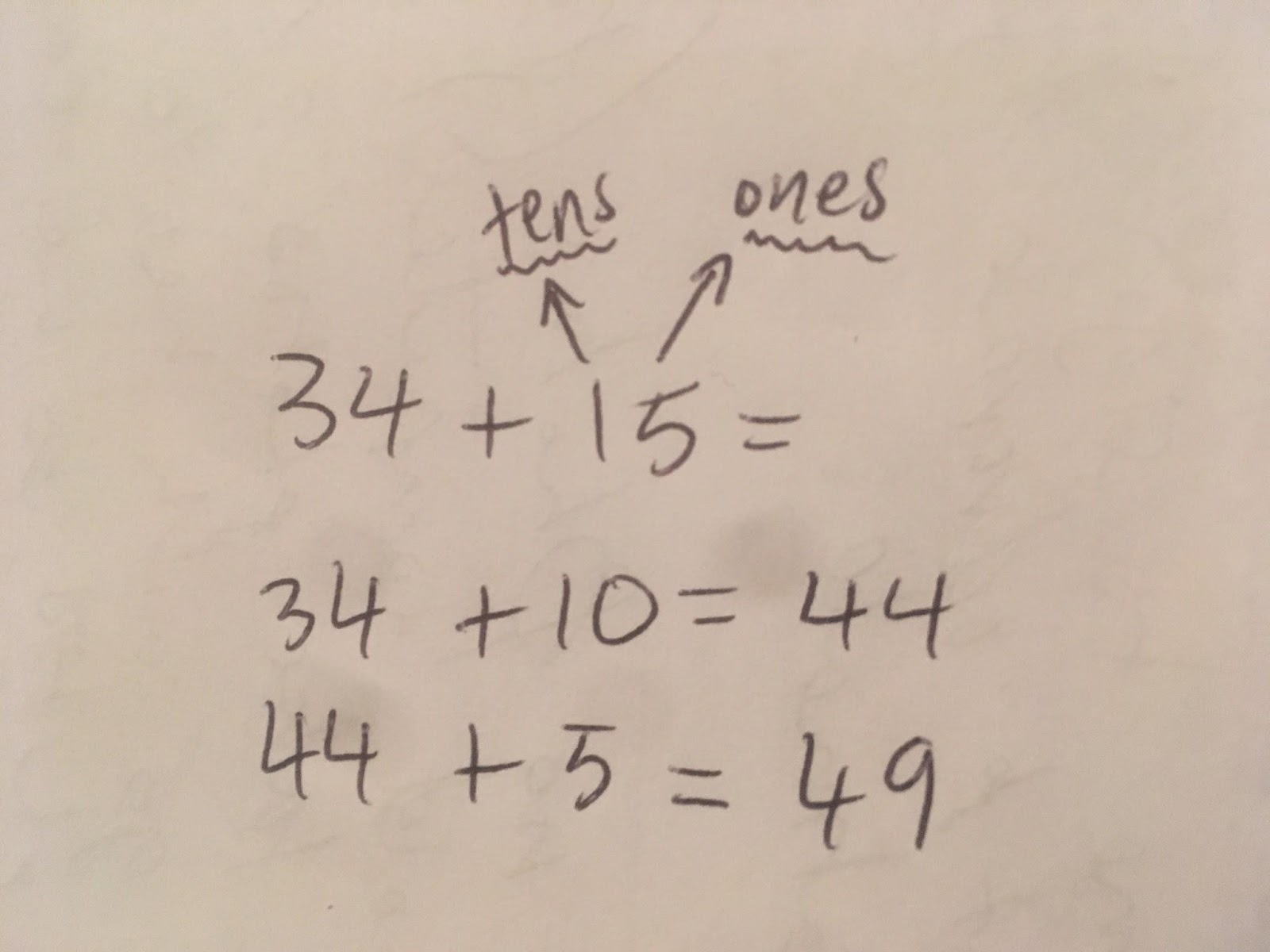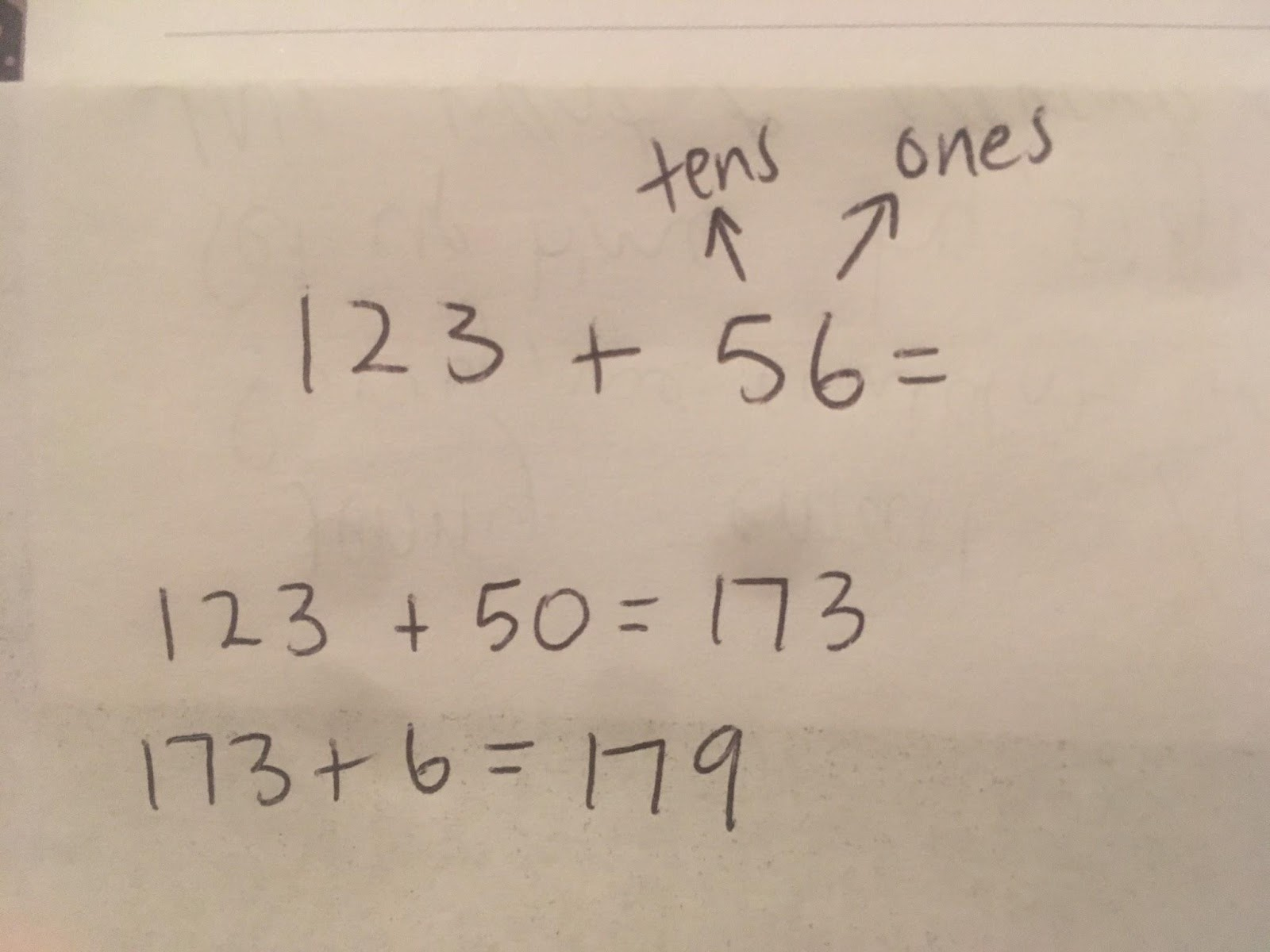## Tuesday, 7 November 2017

### Tha Zin Addition Revision

Add!   Break up the hundreds, tens and ones...

 34 + 1556 + 36 56 + 36 = ? 56 + 30 = 86 86 + 6 = 92 Answer : 92 123 + 5645 + 38 45 + 38 = ? 45 + 30 = 75 75 + 8 = 83 Answer : 83 124 + 69 124 + 69 = ? 120 + 60 = 180 180 + 9 = 189 Answer : 189 91 + 36 91 + 36 = ? 91 + 30 = 121 121 + 6 = 127 Answer : 127 138 + 25 138 + 25 = ? 138 + 20 = 158 158 + 5 = 163 Answer : 163 Pua ate 37 corn chips. Later he ate 75 hot chips. How many chips did he eat that day? 37 + 75 = ? 37 + 70 = 107 107 + 5 = 112 Answer : 112 Sala has 129 Pokemon cards and his brother has 34. How many cards do they have all together? 129 + 34 = ? 129 + 30 = 159 159 + 4 = 163 Answer : 163